# How to Calculate and Solve for Well Bore Storage Factor, Fluid Density and Well Annulus Cross-Sectional Area in Well Testing | The Calculator Encyclopedia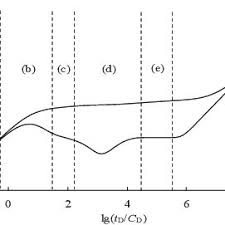The image above represents the well bore storage factor.

To compute for the well bore storage factor, two essential parameters are needed and these parameters are Well Annulus Cross-Sectional Area (Ao) and Fluid Density (ρ).

The formula for calculating the well bore storage factor:

C = 144Ao / 5.615ρ

Where;

C = Well Bore Storage Factor
Ao = Well Annulus Cross-Sectional Area
ρ = Fluid Density

Let’s solve an example;
Find the well bore storage factor when the well annulus cross-sectional area is 54 and fluid density is 42.

This implies that;

Ao = Well Annulus Cross-Sectional Area = 54
ρ = Fluid Density = 42

C = 144Ao / 5.615ρ
C = 144 x 54 / 5.615 x 42
C = 7776/235.83
C = 32.97

Therefore, the well bore storage factor is 32.97.

Calculating the Well Annulus Cross-Sectional Area when the Well bore Storage Factor and the Fluid Density is Given.

Ao = C x 5.615ρ / 144

Where;

Ao = Well Annulus Cross-Sectional Area
C = Well Bore Storage Factor
ρ = Fluid Density

Let’s solve an example;
Find the well annulus cross-sectional area when the well bore storage factor is 34 and fluid density is 24.

This implies that;

C = Well Bore Storage Factor = 34
ρ = Fluid Density = 24

Ao = C x 5.615ρ / 144
Ao = 34 x 5.615 x 24 / 144
Ao = 4581.84 / 144
Ao = 31.81

Therefore, the well annulus cross-sectional area is 31.81.

Calculating the Fluid Density when the Well bore Storage Factor and the Well Annulus Cross-sectional Area is Given.

ρ = 144Ao / C x 5.615

Where;

ρ = Fluid Density
Ao = Well Annulus Cross-Sectional Area
C = Well Bore Storage Factor

Let’s solve an example;
Find the fluid density when the well bore storage factor is 16 and well annulus cross-sectional area is 20.

This implies that;

Ao = Well Annulus Cross-Sectional Area = 16
C = Well Bore Storage Factor = 20

ρ = 144Ao / C x 5.615
ρ = 2304 / 112.3
ρ = 20.51

Therefore, the fluid density is 20.51.

Nickzom Calculator – The Calculator Encyclopedia is capable of calculating the well bore storage factor.

To get the answer and workings of the well bore storage factor using the Nickzom Calculator – The Calculator Encyclopedia. First, you need to obtain the app.

You can get this app via any of these means:

To get access to the professional version via web, you need to register and subscribe for NGN 1,500 per annum to have utter access to all functionalities.
You can also try the demo version via https://www.nickzom.org/calculator

Apple (Paid) – https://itunes.apple.com/us/app/nickzom-calculator/id1331162702?mt=8
Once, you have obtained the calculator encyclopedia app, proceed to the Calculator Map, then click on Petroleum under EngineeringNow, Click on Well Testing under Petroleum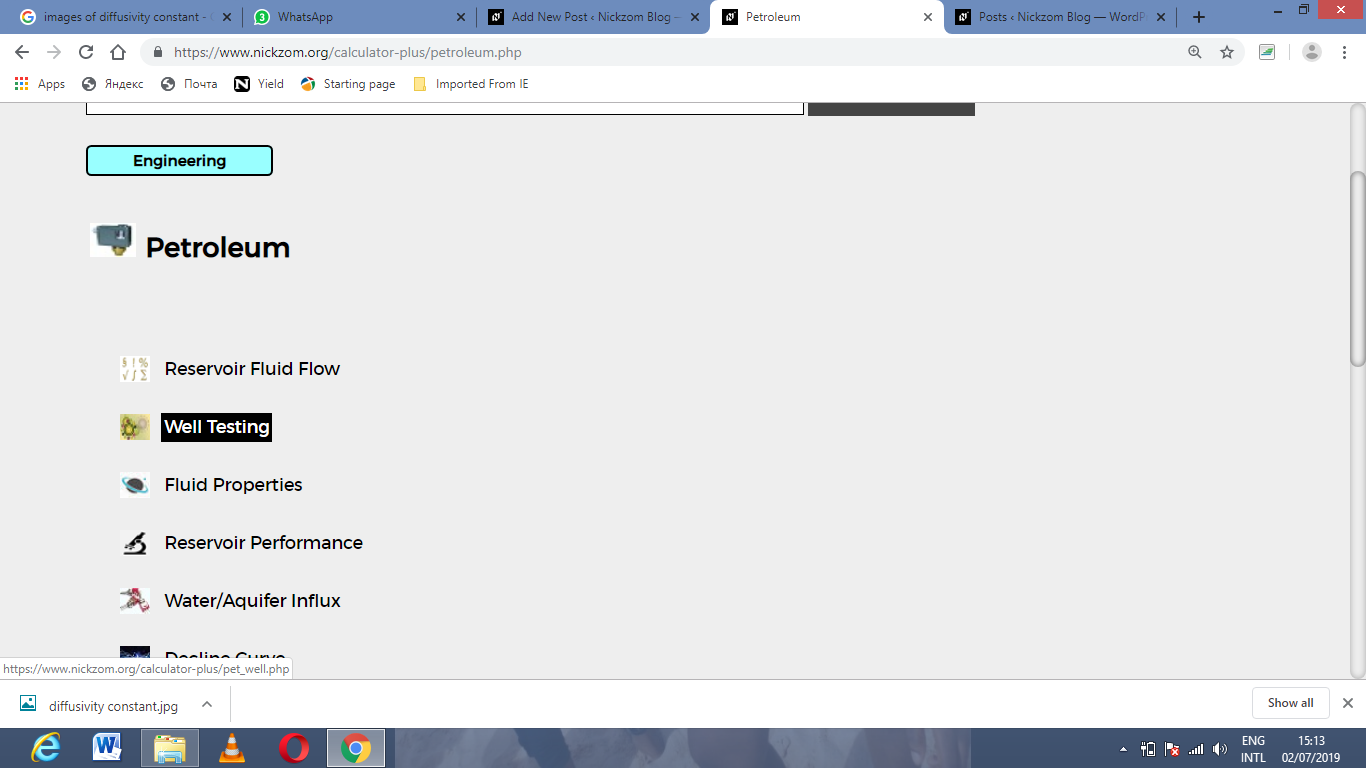Now, Click on Well Bore Storage Factor under Well Testing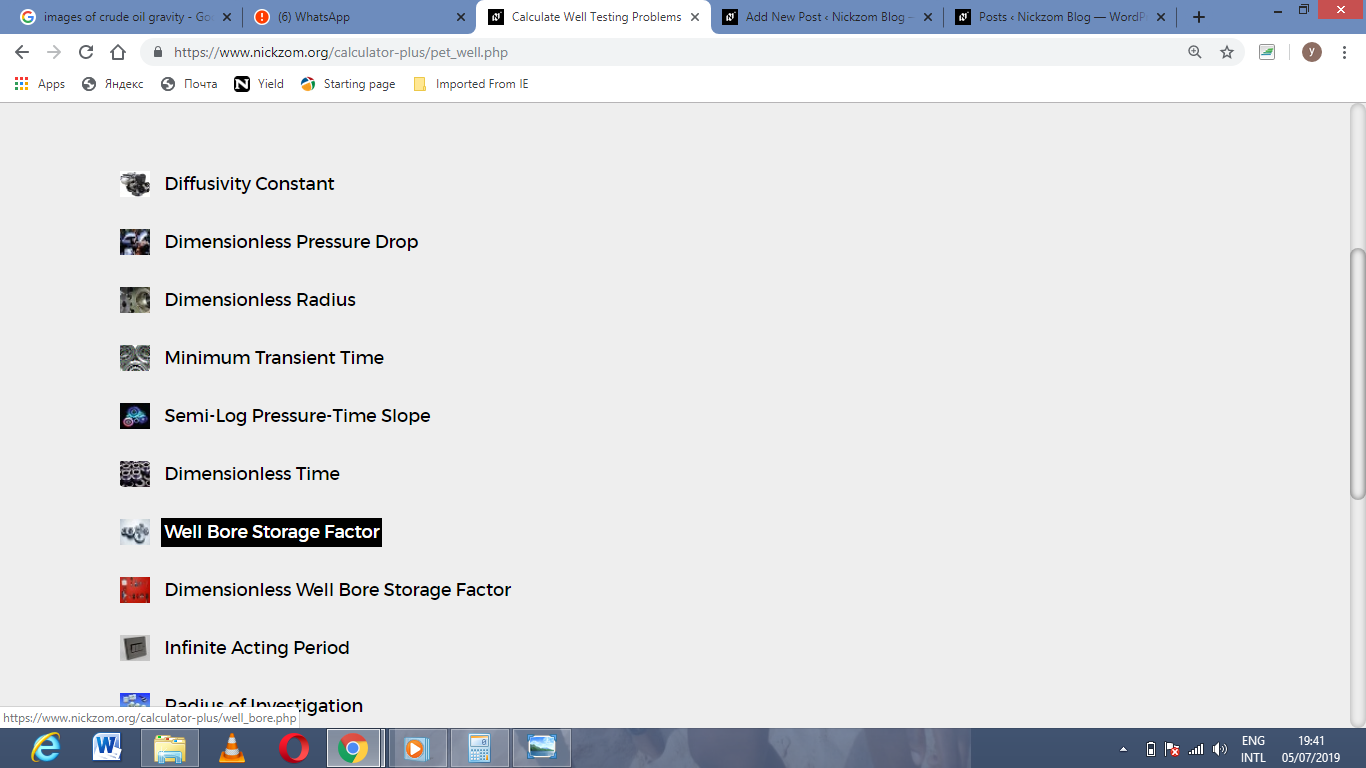The screenshot below displays the page or activity to enter your values, to get the answer for the well bore storage factor according to the respective parameters which are the Well Annulus Cross-Sectional Area (Ao) and Fluid Density (ρ).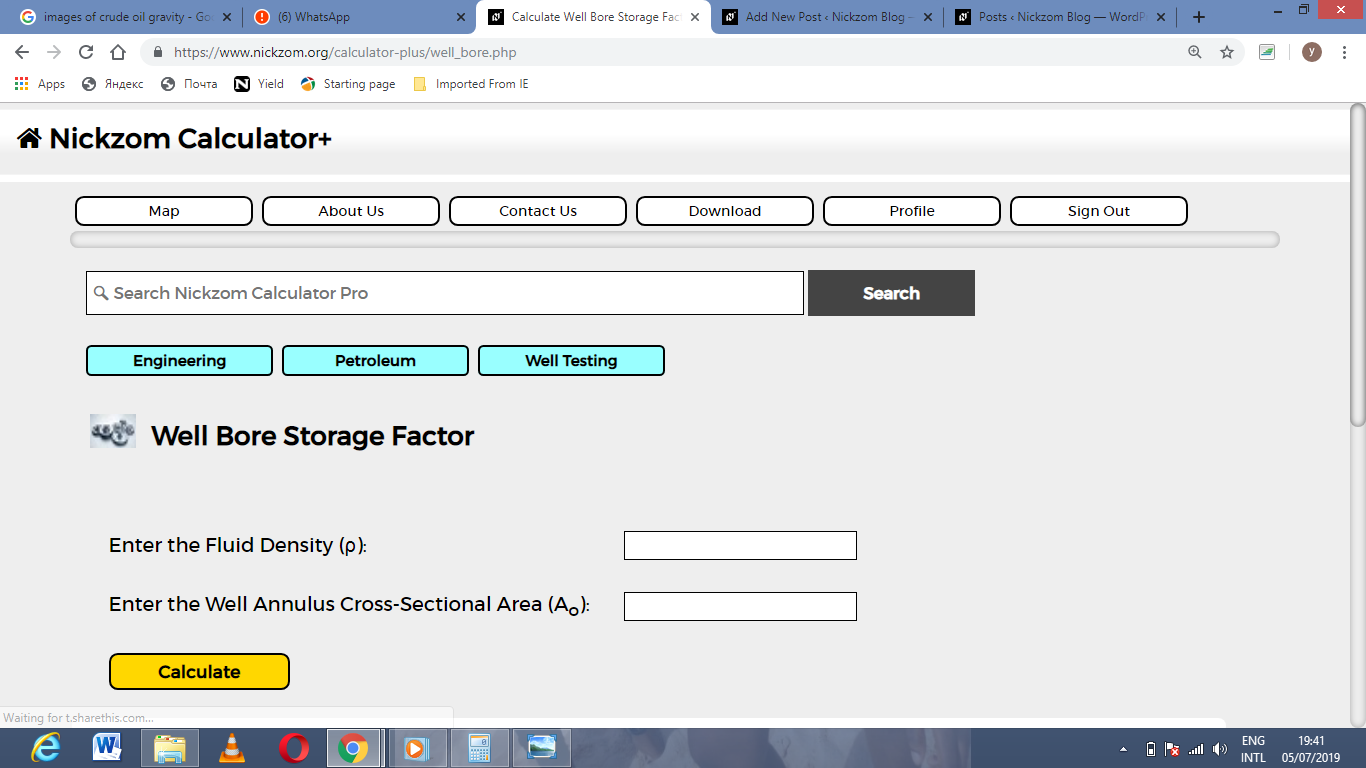Now, enter the values appropriately and accordingly for the parameters as required by the Well Annulus Cross-Sectional Area (Ao) is 54 and Fluid Density (ρ) is 42.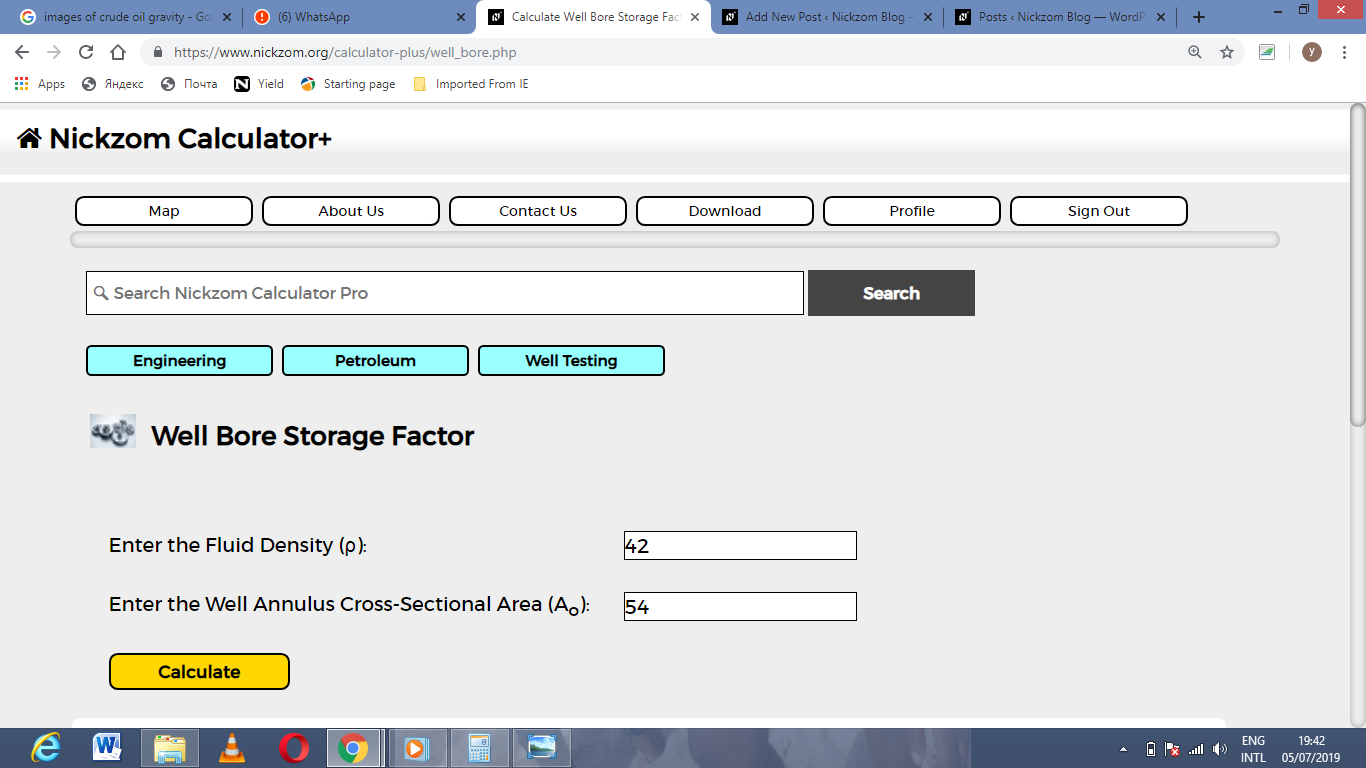Finally, Click on Calculate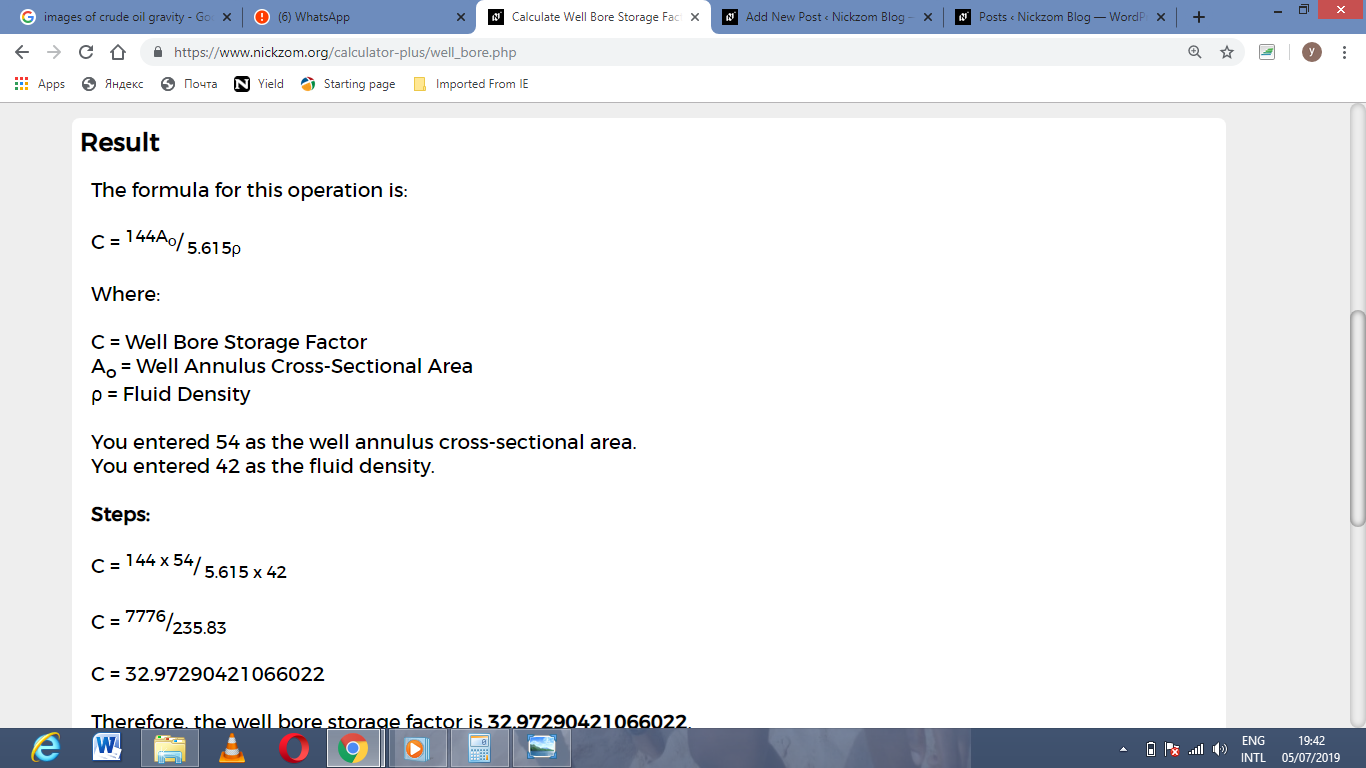As you can see from the screenshot above, Nickzom Calculator– The Calculator Encyclopedia solves for the well bore storage factor and presents the formula, workings and steps too.